#### Cube complex

How is the coordination space represented when there are multiple paths for each robot? It turns out that a cube complex is obtained, which is a special kind of singular complex (recall from Section 6.3.1). The coordination space for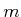fixed paths can be considered as a singular-simplex. For example, the problem in Figure 7.9 can be considered as a singular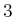-simplex,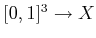. In Section 6.3.1, the domain of a-simplex was defined using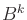, a-dimensional ball; however, a cube,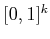, also works becauseandare homeomorphic.

For a topological space,, let a-cube (which is also a singular-simplex),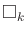, be a continuous mapping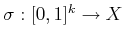. A cube complex is obtained by connecting together-cubes of different dimensions. Every-cube for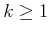has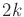faces, which are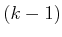-cubes that are obtained as follows. Let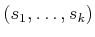denote a point in. For each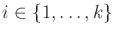, one face is obtained by setting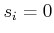and another is obtained by setting.

The cubes must fit together nicely, much in the same way that the simplexes of a simplicial complex were required to fit together. To be a cube complex,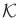must be a collection of simplexes that satisfy the following requirements:

1. Any face,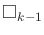, of a cube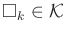is also in.
2. The intersection of the images of any two-cubes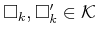, is either empty or there exists some cube,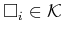for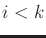, which is a common face of bothand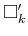.

Let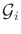denote a topological graph (which may also be a roadmap) for robot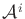. The graph edges are paths of the form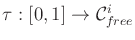. Before covering formal definitions of the resulting complex, consider Figure 7.10a, in which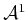moves along three paths connected in a T'' junction and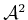moves along one path. In this case, three 2D fixed-path coordination spaces are attached together along one common edge, as shown in Figure 7.10b. The resulting cube complex is defined by three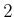-cubes (i.e., squares), one-cube (i.e., line segment), and eight 0-cubes (i.e., corner points).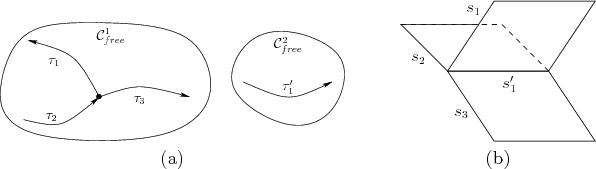Now suppose more generally that there are two robots,and, with associated topological graphs,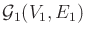and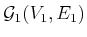, respectively. Suppose that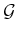and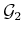have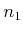and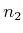edges, respectively. A 2D cube complex,, is obtained as follows. Let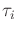denote theth path of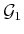, and let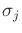denote the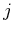th path of. A-cube (square) exists infor every way to select an edge from each graph. Thus, there are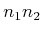-cubes, one for each pair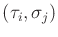, such that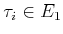and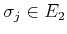. The-cubes are generated for pairs of the form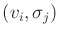for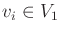and, or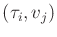forand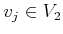. The 0-cubes (corner points) are reached for each pair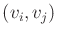such thatand.

If there arerobots, then an-dimensional cube complex arises. Every-cube corresponds to a unique combination of paths, one for each robot. The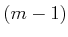-cubes are the faces of the-cubes. This continues iteratively until the 0-cubes are reached.

Steven M LaValle 2020-08-14